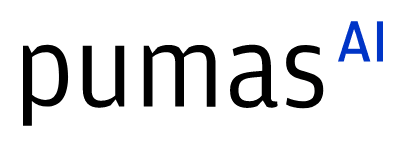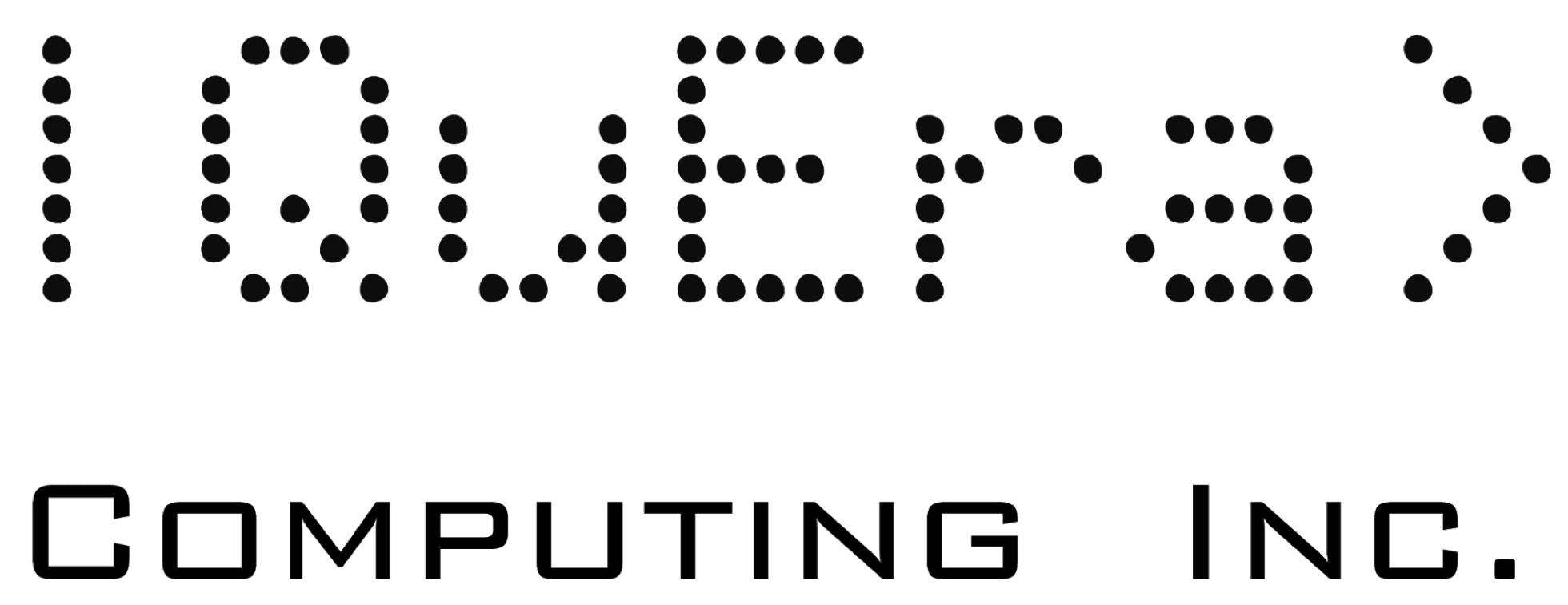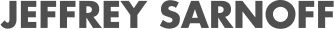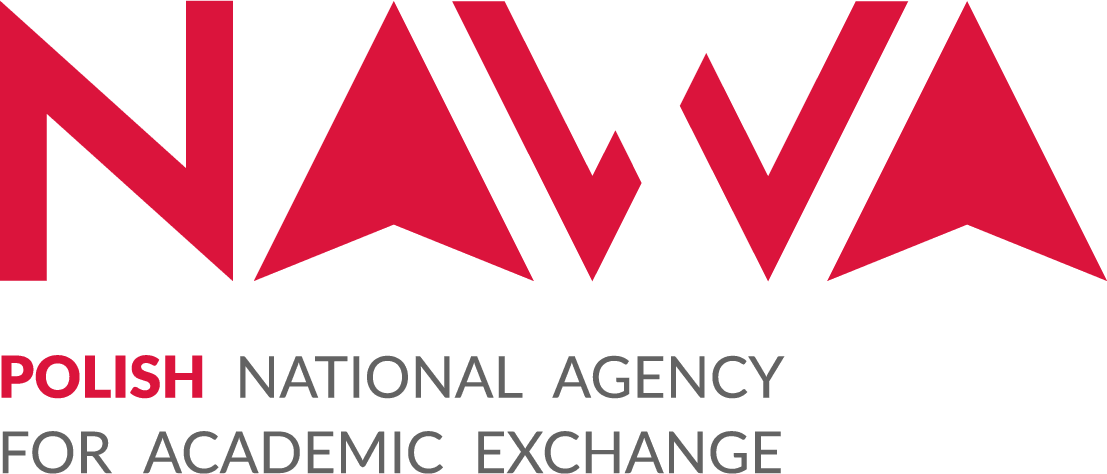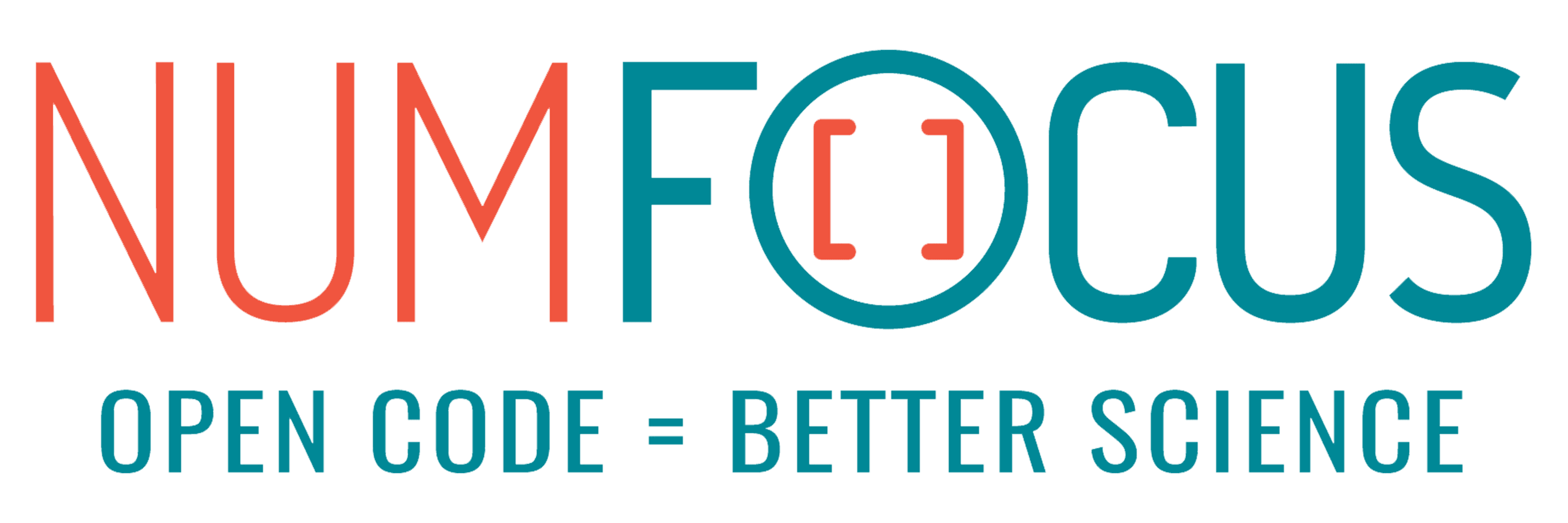# Graph alignment problem within GraphsOptim.jl

07/27/2023, 3:30 PM3:40 PM UTC
32-123

#### Abstract:

Graph alignment is the problem of recovering a bijection between vertex sets of two graphs, that minimizes the divergence between edges. This problem can be encountered in various fields where graphs are often very large, so an efficient algorithm is essential for many applications. In this talk we will discuss how to solve this problem approximately with the popular Fast Approximate Quadratic (FAQ) algorithm and its recent improved variants implemented in the Julia GraphsOptim.jl package.

#### Description:

Graph alignment, also known as graph matching, is the problem of recovering a bijection between the vertex sets of two graphs, that minimizes edge disagreements.

Given the adjacency matrices A,B ∈ 𝐑ᴺˣᴺ of the graphs G₁ and G₂ with vertex set {1, . . ., N} the problem can be formulated as follows:

min ||A−PBPᵀ||²

s.t. P ∈ 𝑷

where 𝑷 is the set of N×N permutation matrices and ||·|| is the Frobenius norm. In the case of two isomorphic graphs its solution is an isomorphism. The calculation of its solution is NP-hard.

The graph alignment problem can be encountered in various fields in which graphs have to be compared such as computer vision, social networks, molecular biology and neuroscience. In the latter, areas of connectomes (nodes of graphs representing neural connections in the brain) are analyzed. These kinds of graphs are often very large, so efficient graph alignment algorithms are essential for many applications. One of the most popular algorithms that solves the problem is the Fast Approximate Quadratic (FAQ) algorithm [1,2]. It is designed to solve a relaxed version of the above formulation, in which the convex-hull of the permutation matrices, i.e. doubly stochastic matrices, is considered as a feasible region. In this way, the Frank–Wolfe algorithm can be applied to find a doubly stochastic matrix, which is subsequently projected by solving a linear assignment problem, onto the set of permutation matrices, finding an approximate solution.The linear assignment problem is also used at each iteration of the Frank-Wolfe algorithm and is the bottleneck of the procedure. For this reason, it has been replaced in the recent GOAT algorithm  with the optimal transport problem solved via the Sinkhorn algorithm. In this talk, we will first briefly introduce the GraphsOptim.jl package  and then focus on the details and Julia implementation of the FAQ and GOAT algorithms.

 J. T. Vogelstein et al., “Fast Approximate Quadratic Programming for Graph Matching,” PLoS ONE, vol. 10, no. 4, p. e0121002, Apr. 2015, doi: 10.1371/journal.pone.0121002.

 https://github.com/microsoft/graspologic

 A. Saad-Eldin, B. D. Pedigo, C. E. Priebe, and J. T. Vogelstein, “Graph Matching via Optimal Transport.” arXiv, Nov. 09, 2021. Accessed: Dec. 21, 2022. [Online]. Available: http://arxiv.org/abs/2111.05366

 https://github.com/gdalle/GraphsOptim.jl